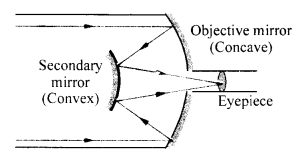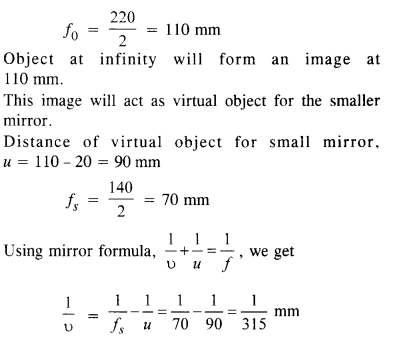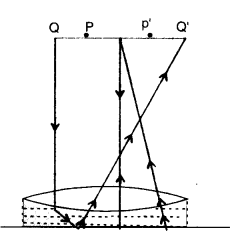NCERT Solutions for Class 12 Physics Chapter 9 Ray Optics and Optical Instruments

NCERT Solutions for Class 12 Physics Chapter 9 Ray Optics and Optical Instruments are part of NCERT Solutions for Class 12 Physics. Here we have given. NCERT Solutions for Class 12 Physics Chapter 9 Ray Optics and Optical Instruments

 Board CBSE Textbook NCERT Class Class 12 Subject Physics Chapter Chapter 9 Chapter Name Ray Optics and Optical Instruments Number of Questions Solved 37 Category NCERT Solutions

Question 1.
A small candle 2.5 cm in size is placed 27 cm in front of a concave mirror of a radius of curvature of 36 cm. At what distance from the mirror should a screen be placed in order to receive a sharp image? Describe the nature and size of the image. If the candle is moved closer to the mirror, how would the screen have to be moved?
Here, h = 2.5 cm, u = – 27 cm, R = – 36 cm.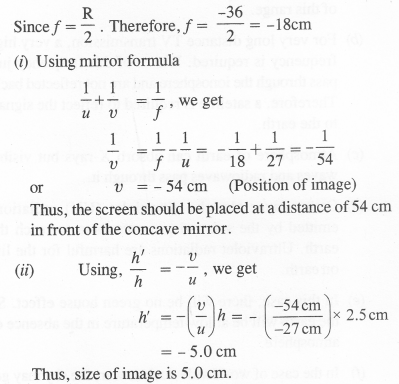Nature of image : Real, inverted and magnified. When the position of the object (i.e. candle) is moved closer to the concave mirror, the distance of the image moves away from the screen till the distance of the candle from the concave mirror is less than 18 cm. Hence, the screen has to be moved away from the concave mirror. When the distance of the candle is less than 18 cm from the concave mirror, a virtual and magnified image of the candle is formed behind the mirror. This image is not obtained on the screen.

Question 2.
A 4.5 cm needle is placed 12 cm away from a convex mirror of focal length 15 cm. Give the location of the image and magnification. Describe what happens as the needle is moved farther from the mirror.
Here, h1 = 4.5 cm, μ = – 12 cm, f= 15 cmThus, the image is virtual, erect and diminished. As we move the needle away from the mirror, the image goes on decreasing in size and moves towards the principal focus on the other side.

Question 3.
A tank is filled with water to a height of 12.5 cm. The apparent depth of the needle lying at the bottom of the tank is measured by a microscope to be 9.4 cm. What is the refractive index of water ? If water is replaced by a liquid of refractive index 1.63 up to the same height, by what distance would the microscope have to be moved to focus on the needle again ? (C.B.S.E. 2009 )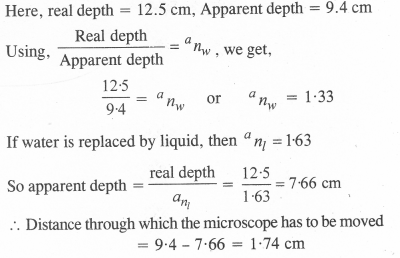Question 4.
The following figures (a) and (b) show refraction of an incident ray in air at 60° with the normal to glass-air and water-air interface, respectively. Predict the angle of refraction of an incident ray in water at 45° with the normal to a water-glass interface [Fig. (c)]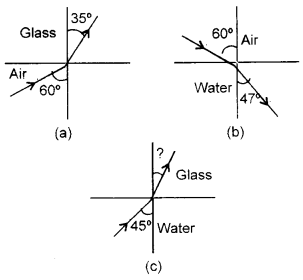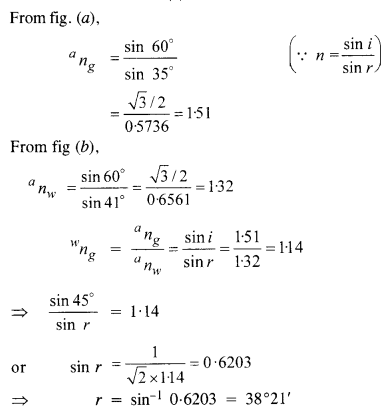Question 5.
A small bulb is placed at the bottom of a tank containing water to a depth of 80 cm. What is the area of the surface of water through which light from the bulb can emerge out ? Refractive index of water is 1.33 (consider the bulb to be a point source).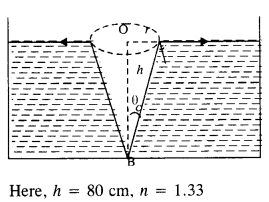The light rays from the bulb B, which fall on the surface of water at an angle equal to critical angle (θC), grazes on the surface of water and the rays of light which fall on the surface of water at an angle greater than θC are totally internally reflected back into the water. The rays of light images emerges out of water through a circular patch of radius r.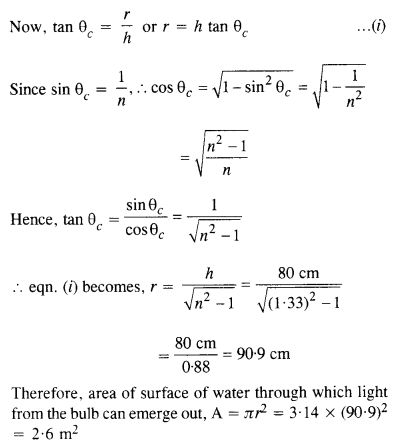Question 6.
A prism is made of glass of unknown refractive index. A parallel beam of light is incident on a face of the prism. By rotating the prism, the angle of minimum deviation is measured to be 40°. What is the refractive index of the material of the prism ? If the prism is placed in water (refractive index 1.33), predict the new angle of minimum deviation of a parallel beam of light. The refracting angle of the prism is 60°.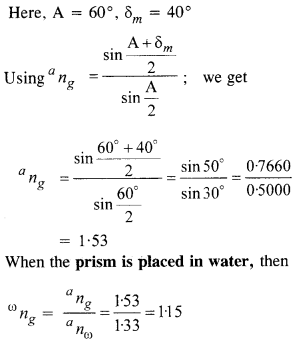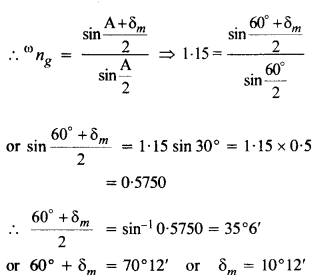Question 7.
Double-convex lenses are to be manufactured from a glass of refractive index 1.55, with both faces of the same radius of curvature. What is the radius of curvature required if the focal length is to be 20 cm ?
Here, n = 1.55, R1 = R and R2 = – R, f= 20 cm Using lens maker formula, we get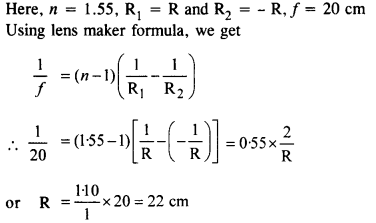Question 8.
A beam of light converges to a point P. A lens is placed in the path of the convergent beam 12 cm from P. At what point does the beam converge if the lens is (a) a convex lens of focal length 20 cm, (b) a concave lens of focal length 16 cm?
(a) When a convex lens is placed in the path of light converging at P, the beam converges at Pt. Thus, point P acts as virtual object for the convex lens.
Now, u = 12 cm, f= 20 cm.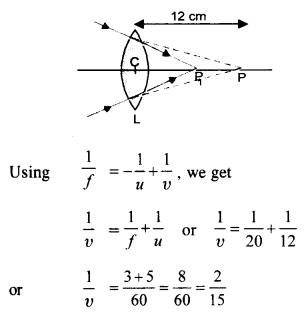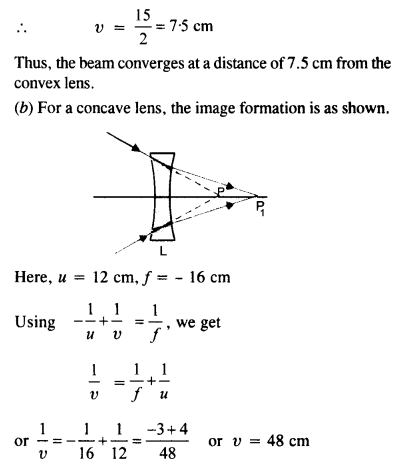Question 9.
An object of size 3.0 cm is placed 14 cm in front of a concave lens of focal length 21 cm. Describe the image produced by the lens. What happens if the object is moved farther from the lens?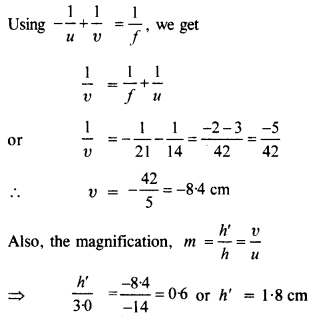Thus, the image is virtual, erect, diminished and is formed on the same side of the lens at a distance of 8.4 cm from the lens. If the object is moved away from the lens, the image moves towards the principal focus and goes on decreasing in size.

Question 10.
What is the focal length of a convex lens of focal length 30 cm in contact with a concave lens of focal length 20 cm? Is the system a converging or a diverging lens? Ignore thickness of the lenses.
Here, f1 = 30 cm and f2 = -20 cm
For the combination of two thin lenses, the focal length of the combination is given by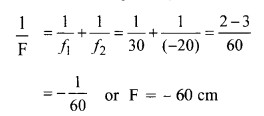Since the focal length of the system of lens is negative, therefore, the combination behaves as a diverging lens.

Question 11.
A compound microscope consists of an objective lens of focal length 2-0 cm and an eye-piece of focal length 6.25 cm separated by a distance of 15 cm. How far from the objective should an object be placed in order to obtain the final image at (a) the least distance of distinct   vision (25 cm), (b) infinity ? What is the magnifying power of the microscope in each case? (C.B.S.E. 2008)
Here, fn =2.0 cm, f = 6.25 cm,
Distance between object lens and eye piece = 15 cm (a) For the formation of image at the least distance of distinct vision,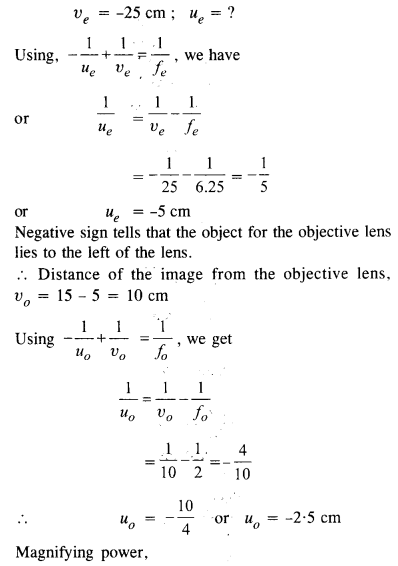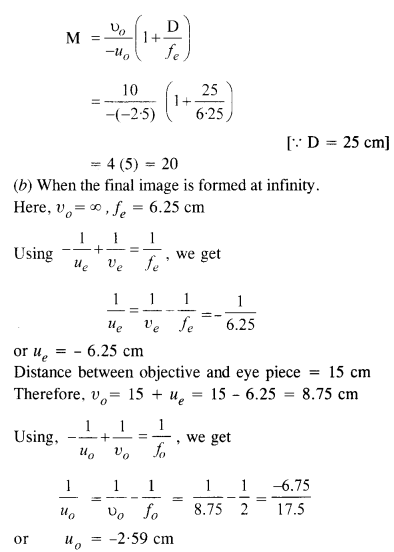Question 12.
A person with a normal near point (25 cm) using a compound microscope with objective of focal length 8.0 mm and an eye-piece of focal length 2.5 cm can bring an object placed 9.0 mm from the objective in sharp focus. What is the separation between the two lenses ? How much is the magnifying power of the microscope?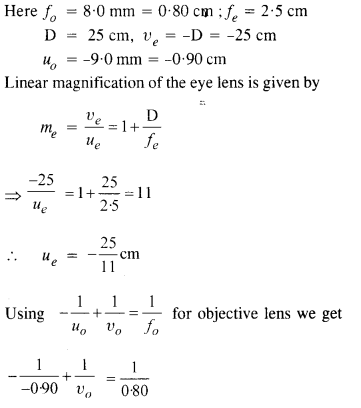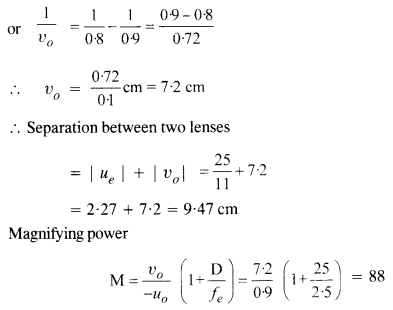Question 13.
A small telescope has an objective lens of focal length 144 cm and an eye-piece of focal length 6.0 cm. What is the magnifying power of the telescope? What is the separation between the objective and the eye-piece?
Here, focal length of objective lens, f0 = 144 cm Focal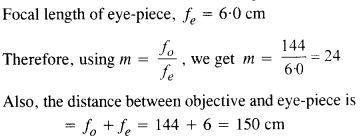Question 14.
(a) A giant refracting telescope at an observatory has an objective lens of focal length 15m. If an eye-piece of focal length 1.0 cm is used, what is the angular magnification of the telescope ?
(b) If this telescope is used to view the moon, what is the diameter of the image of the moon formed by the objective lens ? The diameter of the moon is 3.48 x 106m and the radius of the lunar orbit is 3.8 x 108m. (C.B.S.E. 2008, 2011)Question 15.
Use the mirror equation to deduce that
(a) an object placed between f and 2f of a concave mirror produces a real image beyond 2f.
(b) a convex mirror always produces a virtual image independent of the location of the object.
(c) the virtual image produced by a convex mirror is always diminished in size and is located between the focus and the pole.
(d) an object placed between the pole and focus of a concave mirror produces a virtual and enlarged image. (C.B.S.E. 2011)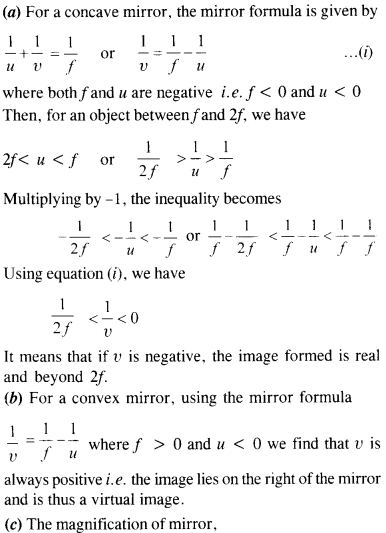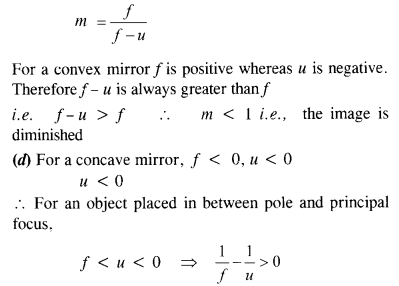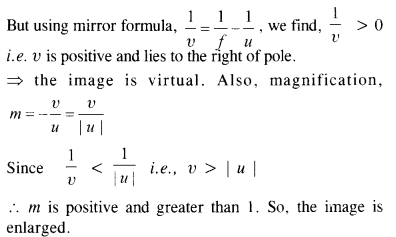Question 16.
A small pin fixed on the table top is viewed from above from a distance of 50 cm. By what distance would the pin appear to be raised if it is viewed from the same point through a 15 cm thick glass slab held parallel to the table?
Refractive index of glass = 1.5. Does the answer depend upon the location of the slab?
Here, t = 15 cm, n = 1.5
The lateral displacement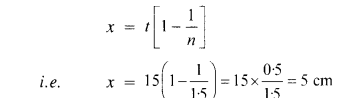For small angles of incidence, the answer does not depend upon the location of the slab.

Question 17.
(a) Following figure shows a cross-section of a ‘light pipe’ made of glass fibre of refractive index 1.68. The outer covering of the pipe is made of material of refractive index 1.44. What is the range of the angles of incident rays with the axes of the pipe for which the total internal reflection inside the pipe take place as shown in the figure ?
(b) What is the answer if there is no outer covering of pipe ?Therefore all incident rays in the range 0 to 90°suffer total internal refletion.

Question 18.

(a) You have learned that plane and convex mirrors produce virtual images of objects. Can they produce real images under some circumstances ? Explain.
(b) A virtual image, we always say, cannot be caught on a screen. Yet when we ‘see’ a virtual image, we are obviously bringing it on to the ‘screen’ (i.e., the retina) of our eye. Is there a contradiction?
(c) A diver under water, looks obliquely at a fisherman standing on the bank of a lake, would the fisherman look taller or shorter to the diver than what he actually is ?
(d) Does the apparent depth of a tank of water change if viewed obliquely ? If so, does the apparent depth increase or decreases
(e) The refractive index of diamond is much greater than that of ordinary glass. Is this fact of some use to a diamond cutter ?
(a) Yes. They can produce real images if the object is a virtual object as shown in figure.(b) here is no contradiction in this case. The virtual image of the object acts as an object for the convex lens of our eye and the lens of our eye make a real image of this object on the ratina.
(c) Let AB be the fisherman standing on the bank of the lake. The rays of light from the head of the fisherman bends towards the normal on refraction at the interface separating water and air. The refracted rays appear to come from point B’ instead of point B for the fish. Thus, for a diver the height of the fisherman is AB’ which is greater than his actual height AB.(d) The apparent depth of a pond of water decreases when viewed obliquely. This is due to the refraction of light from the surface of water.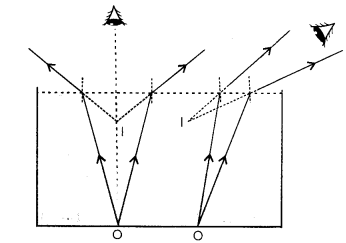Question 19.
The image of a small electric bulb fixed on the wall of a room is to be obtained on the opposite wall 3 m away by means of a large convex lens. What is the maximum possible focal length of the lens required for the purpose ?
The minimum distance between a real object and its real image formed by a convex lens of focal length/is given by L = 4f

Question 20.
A screen is placed 90 cm from an object. The image of the object on the screen is formed by a convex lens at two different locations separated by 20 cm. Determine the focal length of the lens.
Let O be the position of object and I is the position of image when lens is at L1 and then at L2Question 21.
(a) Determine the effective focal length of the combination of the two lenses in the question 910 if they are placed 8.0 cm apart with their principal axes coincident. Does the answer depend on which side a beam of parallel light is incident ? Is the notion of effective focal length of this system useful at all ?
(b) An object 1.5 cm in size is placed on the side of the convex lens in the above arrangements. The distance between the object and the convex lens is 40 cm. Determine the magnification produced by the two-lens system, and the size of the image.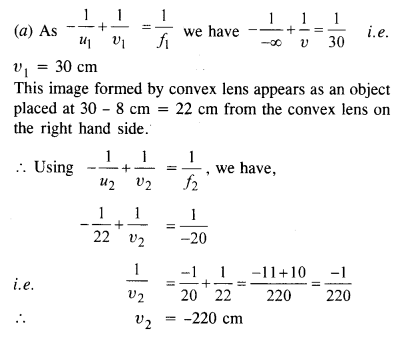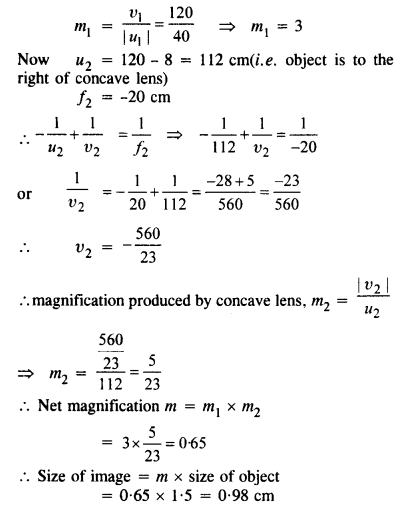Question 22.
At what angle should a ray of light be incident on the face of a prism of refracting angle 60° so that it just suffers total internal reflection at the other face ? The refractive index of the material of the prism is 1.524.
Let the ray of light be incident on the face AB at angle i so that it is totally internally reflected at face AC.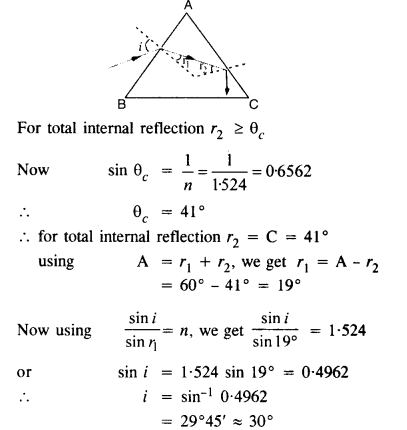Question 23.
You are given prism made of crown glass and flint glass with a wide variety of angles. Suggest a combination of prisms which will (a) deviate a pencil of white light without much dispersion (b) disperse (and displace) a pencil of white light without much deviation.
(a)
Angular dispersion produced by two prisms i.e. crown glass and flint glass should be zero in this caseIn the combination of prisms, flint glass prism of greater angle may be tried but in any case still this angle will be smaller than the angle of the crown glass prism in opposite order as shown in figure.

Question 24.
For a normal eye, the far point is at infinity and the near point of distinct vision is about 2.5 cm in front of the eye. The cornea of the eye provides a converging power of about 40 dioptres, and the least converging power of the eye lens behind the cornea is about 20 dioptres. From this rough data estimate the range of accommodation {i.e., the range of converging power of the eye-lens) of a normal eye.
When the object is placed at infinity, the eye makes use of the least converging power, Therefore, total converging power of cornea and the eye lens = 40 + 20 = 60 dioptre.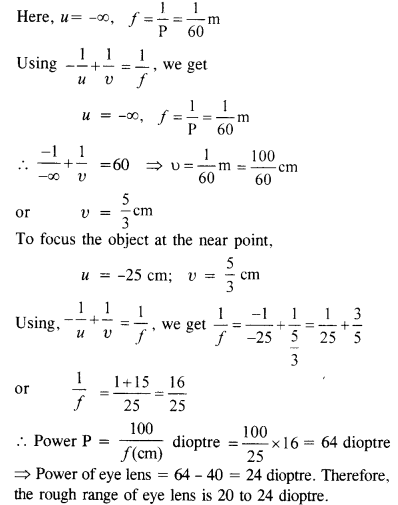Question 25.

Does short-sightedness (myopia) or long-sightedness (hypermetropia) imply necessarily that the eye has partially lost its ability of accommodation ? If not, what might cause these defects of vision ?
No. Myopia may arise due to the elongation of the eye ball and hypermetropia may arise due to the decrease in the size of the eye ball even when the eye has the normal ability of accommodation. There is another defect in the eye called presbyopia similar to hypermetropia. However, the causes of presbyopia and hypermetropia are different. Presbyopia arises in elderly persons and is corrected by using a bi-focal lens.

Question 26.
A myopic person has been using spectacles of power – 1.0 dioptres for distant vision. During old age he also needs to use separate reading glass of power +2 dioptres. Explain what may have happened.
For -1.0 dioptre, the far point for eyes is 1 m i.e. 100 cm. The near point is 25 cm. The objects lying at infinity are brought at 100 cm from his eyes using the concave lens and the objects lying in between 25 cm and 100 cm are brought to focus using the ability of accommodation of the eye lens. In the old age, this ability of accommodation is reduced and the near point reaches 50 cm from his eyes.Question 27.

A person looking at a mesh of crossed wires is able to see the vertical wires more distinctly than the horizontal wires. What is this defect due to ? How is such a defect of vision corrected ?
This is due to the defect of lenses called astigmatism. The defect arises because of the fact that curvature of the eye-lens and the cornea is not same in different planes. This defect is removed by using cylindrical lens with vertical axis.

Question 28.
A man with normal near point (25 cm) reads a book with small print using a magnifing glass: a thin convex lens of focal length 5 cm.
(a) What is the closest and the farthest distance at which he can read the book when viewing through the magnifying glass ?
(b) What is the maximum and minimum angular magnification (magnifying power) possible using the above simple microscope ?
(a) To see the object at a closest distance, the image of object should be formed at the least distance of distinct vision.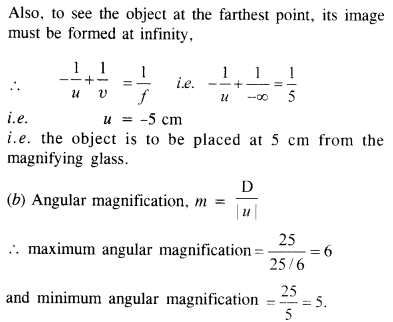Question 29.
A card sheet divided into squares each of size 1 mm2 is being viewed at a distance of 9 cm through a magnifying glass (a converging lens of focal length 10 cm) held close to eye.
(a) What is the magnification (image size/object size) produced by the lens ? How much is the area of each square in the virtual object ?
(b) What is the angular magnification (magnifying powers) of the lens ?
(c) Is the magnification in
(1) equal to magnifying power in
(2) ? Explain.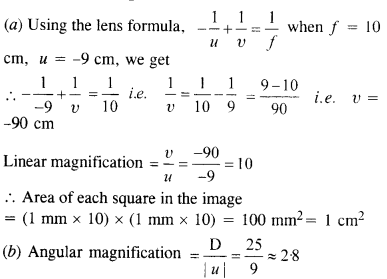(c) Clearly magnification and power magnification are not equal to each other unless the image is located near the least distance of distinct vision, e. v = D.

Question 30.
(a) At what distance should the lens be held from the figure in the above exercise in order to view the squares distinctly with maximum possible magnifying power ?
(b) What is the magnification (image size/object size) in this case ?
(c) Is the magnification equal to magnifying power in this case ? Explain.
(a) The magnifying power is maximum if the image is formed at the least distance of distinct point from the eye, i.e., if υ = -25 cm ; Also, f = 10 cm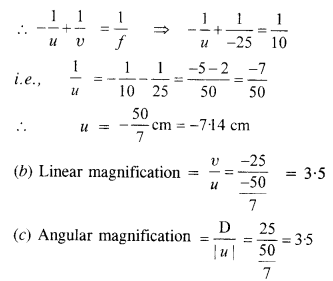=> the linear magnification and magnifying power is equal in this case.

Question 31.
What should be the distance between the object in the previous exercise and the magnifying glass if the virtual image of each square in the figure is to have an area 6.25 mm2? Would you be able to see the squares distinctly with your eyes very close to the magnifier?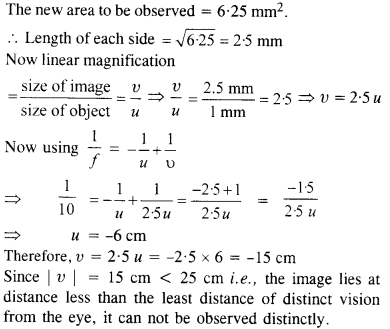Question 32.

(а)
The angle subtended at the eye by an object is equal to the angle subtended at the eye by the virutal image produced by a magnifying glass. In what sense then does a magnifying glass provide angular magnification?
(b) In viewing through a magnifying glass, one usually positions one’s eyes very close to the lens. Does angular magnification change if the eye is moved back?
(c) Magnifying power of a simple microscope is inversely proportional to the focal length of the lens. What then stops us from using a convex lens of smaller and smaller focal length and achieving greater and greater magnifying power?
(d) Why must both the objective and the eye-piece of a compound microscope have short focal lengths?
(e) When viewing through a compound microscope, our eyes should be positioned not on the eye-piece but a short distance away from it for best viewing. Why? How much should be that short distance between the eye and eyepiece?
(a) When magnifying glass is not used, object to be seen clearly is to be placed at 25 cm. However, while using magnifying glass, object can be placed closer to eye than at 25 cm. The closer object has large angular size than the same object placed at 25 cm. It is in this sense that magnifying glass provides angular magnification.
(b) Yes. The angular magnification decreases slightly because angle subtended at eye is somewhat less than the angle subtended at the lens.
(c) The aberrations like spherical and chromatic aberrations start croping up if the convex lens of smaller and smaller focal length is made.
(d) Angular magnification of eye piece is given by (1+D/fe)  and angular magnification of objective is approximately given by υ/f0. Clearly for better magnification focal length of eye piece fe and focal length of objective fe should be small.
(e) If we position our eye very close to the eyepiece, the whole light will not fall on our eye and the field of view will decrease. So we place our eye a short distance away from the eye-piece to collect the large amount of light refracted through the eyepiece to increase the field of view.

Question 33.
An angular magnification (magnifying power) of 30 X is desired using an objective of focal length 1.25 cm and eye piece of focal length 5 cm. How will you set up the compound microscope ?
For the image formed at the least distance of distinct vision, the magnifying power is given by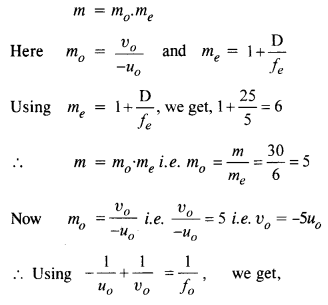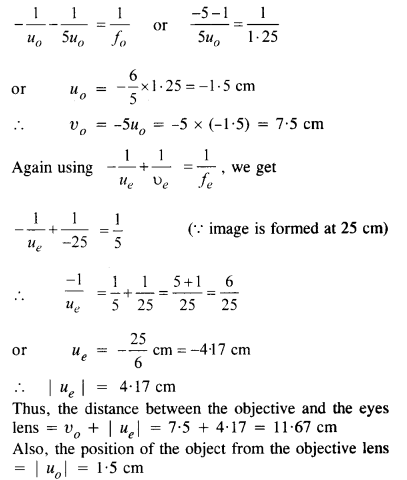Question 34.

A small telescope has an objective lens of focal length 140 cm and an eye piece of focal length 5.0 cm. What is the magnifying power of the telescope for viewing distant objects when
(a) the telescope is in normal adjustment (i.e.,when the final image is formed at infinity) ?
(b) the final image is formed at the least distance of distinct vision (25 cm) ?Question 35.
For the telescope described in the last exercise, in 9.34
(a) what is the separation between the objective lens and the eye-piece ?
(b) If this telescope is used to view a 100 m tall tower 3 km away, what is the height of image of the tower formed by objective lens ?
(c) What is the height of final image of the tower if it is formed at 25 cm ?
(a) Since the final image is formed at infinity, the distance between the object lens and the eye-piece is f0 + fe = 140 + 5 = 145 cm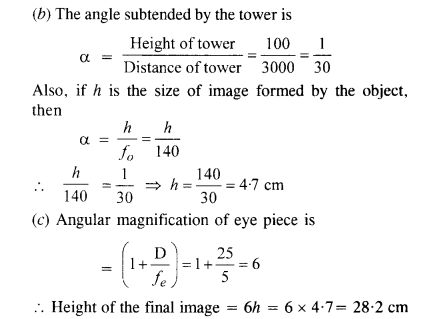Question 36.

A cassegrain telescope uses two mirrors as shown in figure. Such a telescope is built with mirrors 20 mm apart. If the radius of curvature of large mirror is 220 mm and the small mirror is 140 mm, where will be the final image of an object at infinity be ?